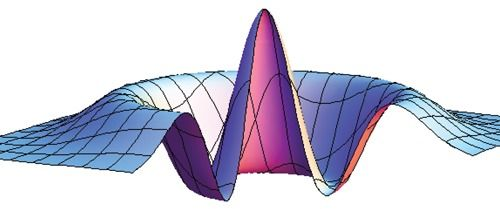## БЛОГNice.

Richard Feynman suggested that it takes a quantum computer to simulate large quantum systems, but a new study shows that a classical computer can work when the system has loss and noise.

The field of quantum computing originated with a question posed by Richard Feynman. He asked whether or not it was feasible to simulate the behavior of quantum systems using a classical computer, suggesting that a quantum computer would be required instead . Saleh Rahimi-Keshari from the University of Queensland, Australia, and colleagues  have now demonstrated that a quantum process that was believed to require an exponentially large number of steps to simulate on a classical computer could in fact be simulated in an efficient way if the system in which the process occurs has sufficiently large loss and noise.

The quantum process considered by Rahimi-Keshari et al. is known as boson sampling, in which the probability distribution of photons (bosons) that undergo a linear optical process  is measured or sampled. In experiments of this kind [4, 5], NN single photons are sent into a large network of beams splitters (half-silvered mirrors) and combined before exiting through MM possible output channels. The calculation of the probability distribution for finding the photons in each of the MM output channels is equivalent to calculating the permanent of a matrix. The permanent is the same as the more familiar determinant but with all of the minus signs replaced with plus signs.